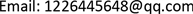1. 背景知识1.1. 背景介绍

1.2. 研究意义

2. 问题的分析2.1. 对环境温度为75℃、II层厚度为6 mm、IV层厚度为5 mm，研究人皮肤外侧的温度分布

2.2. 当环境温度为65℃、IV层的厚度为5.5 mm时，确定II层的最优厚度， 确保工作60分钟时，不超过人体承受范围

2.3. 当环境温度为80℃时，确定II层和IV层的最优厚度，确保工作30分钟时， 假人皮肤外侧温度不超过47℃，且超过44℃的时间不超过5分钟

1、假设热传导是唯一的传热方式；

2、假设材料的厚度为其特征尺寸；

3、织物材料无褶皱且表面温度处处相等；

4、假设温度传递只存在一维传递且沿着温度梯度方向；

5、假设防护服的表面积与人体的表面积相等，均为1.6 m2

6、假设材料的热传导率与表面传感系数在数值上相等；

7、假设不存在导热热阻；

8、假设第III、IV层温度为衣服温度 y 0 = 30 ˚ C ；第I、II层温度为体内温度 y 体 = 37 ˚ C 。

3. 模型的建立与求解3.1. 环境温度75℃时，关于服装温度变化的研究3.1.1. 研究思路

3.1.2. 研究方法

1) 模型原理

Q = λ Δ T d

2) 模型建立

Q = λ 1 y 1 − y ∞ d 1 = λ 2 y 2 − y 1 d 2 = λ 3 y 3 − y 2 d 3 = λ 4 y 4 − y 3 d 4

3) 模型数据处理

y 1 = 71 ˚ C

y 2 = 62.13 ˚ C

y 3 = 57.77 ˚ C

1) 模型的建立

y − y ∞ y 0 − y ∞ = exp ( − h A ρ c V x )

ρ ——表示传热物质密度；

①方程的整理：

y = ( y 0 − y ∞ ) exp ( − g h A ρ c V x ) + y ∞

y 1 = ( y 0 − y ∞ ) exp ( − 1.35 h A ρ c V x ) + 75

y 2 = ( y 0 − y 1 ) exp ( − 10.44 h A ρ c V x ) + y 1

y 3 = ( y 体 − y 2 ) exp ( − 3.95 h A ρ c V x ) + y 2

y 4 = ( y 体 − y 3 ) exp ( − 0.15 h A ρ c V x ) + y 3

3.2. 环境温度65℃时，第二层最优厚度的研究3.2.1. 研究思路

3.2.2. 研究方法

1) 模型准备

2) 模型的建立

m < 12   kg

m = m I + m II + m III + m IV

0.0006   m < d 2 < 0.025   m

y 21 = 65 ˚ C

λ 1 y 1 − y 2 d 1 > λ 2 y 21 − y 22 d 21

y 21 − y 22 < 8.87

g 4 = 0.15

λ 1 y 21 − y 22 d 1 = λ 2 y 22 − y 23 d 2 = λ 3 y 23 − y 24 d 3 = λ 4 y 23 − 47 d 4

44 ≥ ( y 体 − y x = 55 ) exp ( − g 4 h A ρ c V ) + y x = 55

47 ≥ ( y 体 − y 23 ) exp ( − g 4 h A ρ c V ) + y 23

y x = 55 > 44 ˚ C

y 23 > 47 ˚ C

3) 模型的求解

Temperature form of each layer in 60 minute

60分钟65℃56.13℃48.09℃44.77℃

3.3. 当环境温度为80℃，确定II层和IV层的最优厚度3.3.1. 研究思路

3.3.2. 研究方法

1) 模型原理与建立

∂ y i ∂ x i = λ i c i ρ i ∂ 2 y i ∂ l 2

λ i ∂ y i ∂ x = λ i + 1 ∂ y i + 1 ∂ x , y i = y i + 1 , l = d i , ( i = 1 , 2 , 3 , 4 )

{ ∂ y i ∂ x = λ i c i ρ i ∂ 2 y i ∂ l 2 λ i ∂ y i ∂ l = λ i + 1 ∂ y i + 1 ∂ l , y i = y i + 1 , l = d i , ( i = 1 , 2 , 3 ) y 0 ( 0 , x ) = 80 ˚ C y 4 ( L 4 , x ) = y 0 = 37 ˚ C

2) 模型求解

x——时间；d(Y)——厚度(空间变量)；U——温度；

4. 结论